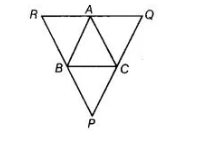# Through A, B and C lines RQ,`
Question:

Through A, B and C lines RQ, PR and QP have been drawn, respectively parallel to sides BC, CA and AB of a ΔABC as shown in figure. Show that  BC = ½ QRSolution:

Given In ΔABC, PQ || AB and PR || AC and RQ || BC.

To show BC = ½ QR

Proof In quadrilateral BCAR, BR || CA and BC|| RA

So, quadrilateral, BCAR is a parallelogram.

BC = AR …(i)

Now, in quadrilateral BCQA, BC || AQ

and AB||QC

So, quadrilateral BCQA is a parallelogram,

BC = AQ …(ii)

On adding Eqs. (i) and (ii), we get

2 BC = AR+ AQ

=> 2 BC = RQ

=> BC = ½ QR

Now, BEDF is a quadrilateral, in which ∠BED = ∠BFD = 90°

∠FSE = 360° – (∠FDE + ∠BED + ∠BFD) = 360° – (60° + 90° + 90°)

= 360°-240° =120°